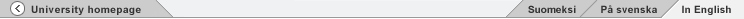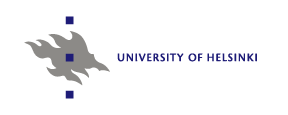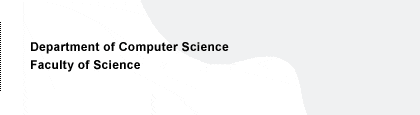# 582450 Modeling of Vision

## Practical work for the 1st half

The following will be done on Octave, which is a free Matlab clone. You can also use Matlab if you really want to. Here is some introductory documentation on Octave.

Important notice concerning octave versions: There are different versions of octave around. We recommend you use (the slightly outdated) version 2.0.17, started on UH-CS machines by /opt/octave-2.0.17/bin/octave, because it should the most compatible with the other software used below.

1. Find a gray-scale image somewhere (on the web, for example). It must be sufficiently large (say, at least 300x300). WARNING: Try to find images that have not bern compressed, compression can induce very nasty effects. You can also take a colour image and convert it to grayscale using xv or other software. To load the image into Octave or Matlab, you may find the command imread useful. To use it in Octave 2.0.17, you need to download it here. Some other versions of octave may have it installed.
2. Take small patches (16x16) of the image. 10,000 is a sufficient amount of patches. Here's some code to do the sampling
3. Design three different Gabor receptive fields, using at three different orientations and frequencies. Try to make the parameters so that the receptive fields are realistic models of the V1 simple cells (but the size of the RF's does not matter). Plot the RF's.
4. Compute the outputs of the RF's for the image patches. Normalize them to unit variance. Plot histograms of the outputs. Compute their kurtoses.
5. Do ICA on the image patches using the FastICA package. The octave 2.0.17 implementation is available here as a tar file. The octave 2.1.55 implementation is available here. The Matlab implementation is here.
6. Plot histograms of the independent components. Compute their kurtoses. Compare the kurtoses with those obtained by the Gabor filters. Plot (some of the) RF's. Look at the RF's and comment on whether they look like V1 RF's or not. If not, why not?

And finally: write a report of approx. 3 pages. The approximate contents of the report is as follows. One page is for figures containing the results (figures). One is for the Octave/Matlab code, with comments, specify which software you are using. One page is for comments on the results and explanation of the choice of parameters etc. Return this report (a single file in PS or PDF format) to Urs Köster (email below).

The deadline for the work is is 31th March 2008.

If you have problems, contact Urs Köster, email urs.koster at cs.helsinki.fi

Aapo Hyvärinen, Jan 2008